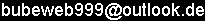Calculator for Windows

Size
packed
Programcategory OS Hardwarerequirements
150kB Tool Windows95 and higher minimal

## Basic concept

After starting the program make your input. After pressing the ENTER-key the result will be shown in a new line. It is possible to edit all your input and the results like one do it usually in text-editors.

Examples:
5+8
13

sin(pi/2)
1

2*3^(38/27)-5.3
4.08715488814264

## Funktions and constants

Calculator for Windows defines all usual functions and constants. For an overview see here. If you select a certain item in the menu, you can see an information for this item in the status-bar of the program. To input a certain function or constant it is not necessary to use the menu, the best way is do type it.

Examples:
arcsin(0.5)/ln(20)
0.174781565168748

exp(3)*h*e/me
2.3407828483984E-21

In addition to this pre-defined mathematical and physical constants it is possible to define user-constans.

## The status bar

The status bar of Calculator for Windows shows you
+ the result in user defined output format
+ the result in standard format
+ the angular mode (Rad, Deg, Grad); click on this cell to change the mode
+ help messages, if move the mouse over special objects like some menu items or status bar cells.

## Set the output format

By default Calculator for Windows types out the results in a standard number format. The output is decimal an with maximal precision; very big and very small numbers are typed out in scientific notation. However it is possible to set other output formats. Just choose the menu Extras|Output format or click on the very left status bar field, to open an option form where you can choose certain output formats and the precision (e.g. number of digits). Output formats can be set for the text field (e.g. the cfw editor window) and for the status bar output.

Calculator for Windows can process hexadecimal and binary number. For output in such a format, choose this format in the output format options. For input use \$ for hexadecimal and # for binary numbers. (Examples: \$ff03, #00111010).

+,-,*,/
^: power
root
(,): brackets
sqrt(...)
cos(...)
sin(...)
tan(...)
ln(...)
exp(...)
log(...)
arcsin(...)
arccos(...)
arctan(...)
rnd(...)
factorial(...)
sinh(...)
cosh(...)
tanh(...)
arsinh(...)
arcosh(...)
artanh(...)
abs(...)
AND
OR

## Build in constants

mathematical:
pi: circle constant
exp(1): Euler's constant

physical:
c: Speed of light
e: Elementary charge
me: Electron mass
h: Planck constant
hbar: h / (2*pi)
kB: Boltzmann constant
mu0: Permeability of vacuum
epsilon0: Permittivity of vacuum
G: Gravitational constant
g: Acceleration due to gravity

conversion:
Celsius0: temperature: 0°C in Kelvin
MeterPerInch: length: Meter / Inch conversion
MeterPerMile: length: Meter / Mile conversion
LiterPerGalone: volume: Liter / Galone conversion
WattPerPS: power: Watt / PS conversion
PascalPerBar: pressure: Pascal / Bar conversion
PascalPerTorr: pressure: Pascal / Torr,mmHg conversion
JoulePerCal: energy: Joule / Cal conversion
TeslaPerGauss: magnetic field: Tesla / Gauss conversion
TeslaPerOersted: magnetic field: Tesla / Oersted conversion

questions and remarks to-> Back